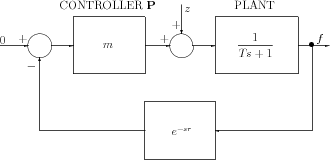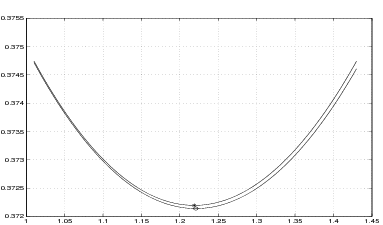]> 5.4.1 Example 1: A simple time–delay control system

#### 5.4.1 Example 1: A simple time–delay control system

Consider the control system containing a time delay in the feedback loop, depicted in Figure 5.1. The disturbance $z$ has a form of the Dirac $\delta$–pseudofunction.Figure 5.1: The automatic control system with a time lag

We wish to solve the parametric optimization problem of ﬁnding minimum of the function

$J\left(m\right)={∥f∥}_{{\text{L}}^{2}\left(0,\infty \right)}^{2}$

where the function $f$ is given by its Laplace transform

$\stackrel{̂}{f}\left(s\right)=\frac{1}{s+\frac{1}{T}+\frac{\beta }{T}{e}^{-sr}},\phantom{\rule{2em}{0ex}}s\in S,$

and $S$ is the stability region in the space of the proportional controller gain $m$,

$S=\left(-1,-\frac{1}{cos{\Omega }_{0}}\right).$

Here ${\Omega }_{0}$ is the smallest positive solution of the equation

$-\frac{r}{T}\phantom{\rule{3.26212pt}{0ex}}\Omega =tan\Omega .$

The explicit exact expression for the performance index as a function of $m$ is given in ,

 ${T}^{2}J\left(m\right)={T}^{2}{∥f∥}_{{\text{L}}^{2}\left(0,\infty \right)}^{2}=\left\{\begin{array}{cc}\frac{msin\kappa r+\kappa T}{2\kappa \left(1+mcos\kappa r\right)},\hfill & \phantom{\rule{1em}{0ex}}{m}^{2}\ge 1\hfill \\ \frac{msinh\kappa r+\kappa T}{2\kappa \left(1+mcosh\kappa r\right)},\hfill & \phantom{\rule{1em}{0ex}}{m}^{2}<1\hfill \end{array}\right\}$ (5.11)

where $\kappa =\frac{1}{T}\sqrt{\left|{m}^{2}-1\right|}$.

Exercise 5.4.1. Conﬁrm (5.11) using the method presented in Section 3.2.

For $T=1$ and $r=0.5$ the exact values of the performance index calculated from (5.11) are compared in Figures 5.2 with the results derived by applying the approximate method presented in Sections 5.2 and 5.3. The lower curve of Figure 5.2 has been obtained by plotting ${∥{P}_{n}f∥}_{{\text{L}}^{2}\left(0,\infty \right)}^{2}$ versus $m$. This was achieved by implementing formula (5.4), with $A$, $\stackrel{̂}{f}\left(-{A}^{\ast }\right)$ and $H$ given by (5.6) and (5.7), on Matlab 6. The ill–conditioning was observed for large $n$, however $n=32$–dimensional approximation ensures satisfactory results. The optimal value obtained by the approximation method is $0.372138$ while by the exact value is $0.372194$. The approximate value of optimal $m$ is $m=1.220738$ while the exact one is $1.218772$. The relative error of ﬁnding the optimal value of $m$ is less than $0.17%$.Figure 5.2: Plots of the performance index for the system examined in Example 1